# 【翻译】Sklearn与TensorFlow机器学习实用指南 —— 第16章 强化学习（下）

+关注继续查看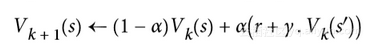a是学习率（例如 0.01）

TD 学习与随机梯度下降有许多相似之处，特别是它一次处理一个样本的行为。就像 SGD 一样，只有当你逐渐降低学习速率时，它才能真正收敛（否则它将在极值点震荡）。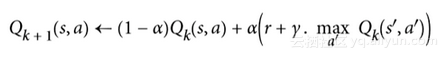import numpy.random as rnd
learning_rate0 = 0.05
learning_rate_decay = 0.1
n_iterations = 20000
s = 0 # 在状态 0开始
Q = np.full((3, 3), -np.inf)  # -inf 对应着不可能的动作
for state, actions in enumerate(possible_actions):
Q[state, actions] = 0.0  # 对于所有可能的动作初始化为 0.0
for iteration in range(n_iterations):
a = rnd.choice(possible_actions[s])  # 随机选择动作
sp = rnd.choice(range(3), p=T[s, a]) # 使用 T[s, a] 挑选下一状态
reward = R[s, a, sp]
learning_rate = learning_rate0 / (1 + iteration * learning_rate_decay)
Q[s, a] = learning_rate * Q[s, a] + (1 - learning_rate) * (reward + discount_rate * np.max(Q[sp]))
s = sp # 移动至下一状态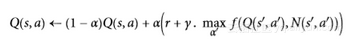N计算了在状态s时选择动作a的次数
f是一个探索函数，例如f=q+K/(1+n)，其中K是一个好奇超参数，它测量智能体被吸引到未知状态的程度。

Q 学习的主要问题是，它不能很好地扩展到具有许多状态和动作的大（甚至中等）的 MDP。试着用 Q 学习来训练一个智能体去玩 Ms. Pac-Man。Ms. Pac-Man 可以吃超过 250 粒粒子，每一粒都可以存在或不存在（即已经吃过）。因此，可能状态的数目大于 2 的 250 次幂，约等于 10 的 75 次幂（并且这是考虑颗粒的可能状态）。这比在可观测的宇宙中的原子要多得多，所以你绝对无法追踪每一个 Q 值的估计值。

$brew install cmake boost boost-python sdl2 swig wget  在 Ubuntu 上，输入以下命令（如果使用 Python 2，用 Python 替换 Python 3）： $ apt-get install -y python3-numpy python3-dev cmake zlib1g-dev libjpeg-dev\    xvfb libav-tools xorg-dev python3-opengl libboost-all-dev libsdl2-dev swig


\$ pip3 install --upgrade 'gym[all]'


>>> env = gym.make("MsPacman-v0")
>>> obs = env.reset()
>>> obs.shape  # [长，宽，通道]
(210, 160, 3)
>>> env.action_space
Discrete(9)


mspacman_color = np.array([210, 164, 74]).mean()

def preprocess_observation(obs):
img = obs[1:176:2, ::2] # 裁剪
img = img.mean(axis=2) # 灰度化
img[img==mspacman_color] = 0 # 提升对比度
img = (img - 128) / 128 - 1 # 正则化为-1到1.
return img.reshape(88, 80, 1)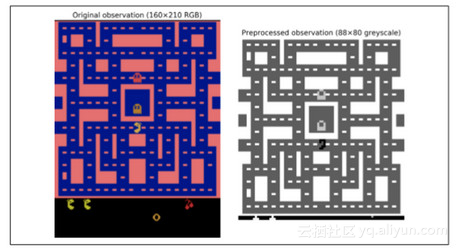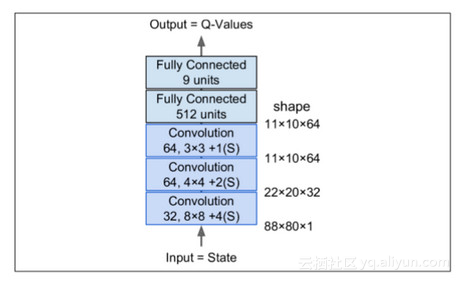from tensorflow.contrib.layers import convolution2d, fully_connected
input_height = 88
input_width = 80
input_channels = 1
conv_n_maps = [32, 64, 64]
conv_kernel_sizes = [(8,8), (4,4), (3,3)]
conv_strides = [4, 2, 1]
conv_activation = [tf.nn.relu]*3
n_hidden_in = 64 * 11 * 10  # conv3 有 64 个 11x10 映射
each n_hidden = 512
hidden_activation = tf.nn.relu
n_outputs = env.action_space.n  # 9个离散动作
initializer = tf.contrib.layers.variance_scaling_initializer()

def q_network(X_state, scope):
prev_layer = X_state
conv_layers = []
with tf.variable_scope(scope) as scope:
for n_maps, kernel_size, stride, padding, activation in zip(conv_n_maps,                                                                  conv_kernel_sizes,
conv_strides,
prev_layer = convolution2d(prev_layer,
num_outputs=n_maps,
kernel_size=kernel_size,
activation_fn=activation,
weights_initializer=initializer)
conv_layers.append(prev_layer)
last_conv_layer_flat = tf.reshape(prev_layer, shape=[-1, n_hidden_in])
hidden = fully_connected(last_conv_layer_flat, n_hidden,
activation_fn=hidden_activation,                                                  weights_initializer=initializer)
outputs = fully_connected(hidden, n_outputs,
activation_fn=None,
weights_initializer=initializer)

trainable_vars = tf.get_collection(tf.GraphKeys.TRAINABLE_VARIABLES,
scope=scope.name)
trainable_vars_by_name = {var.name[len(scope.name):]: var
for var in trainable_vars}
return outputs, trainable_vars_by_name


trainable_vars_by_name字典收集了所有 DQN 的可训练变量。当我们创建操作以将评论家 DQN 复制到行动者 DQN 时，这将是有用的。字典的键是变量的名称，去掉与范围名称相对应的前缀的一部分。看起来像这样：

>>> trainable_vars_by_name
{'/Conv/biases:0': <tensorflow.python.ops.variables.Variable at 0x121cf7b50>, '/Conv/weights:0': <tensorflow.python.ops.variables.Variable...>,
'/Conv_1/biases:0': <tensorflow.python.ops.variables.Variable...>, '/Conv_1/weights:0': <tensorflow.python.ops.variables.Variable...>, '/Conv_2/biases:0': <tensorflow.python.ops.variables.Variable...>, '/Conv_2/weights:0': <tensorflow.python.ops.variables.Variable...>, '/fully_connected/biases:0': <tensorflow.python.ops.variables.Variable...>, '/fully_connected/weights:0': <tensorflow.python.ops.variables.Variable...>, '/fully_connected_1/biases:0': <tensorflow.python.ops.variables.Variable...>, '/fully_connected_1/weights:0': <tensorflow.python.ops.variables.Variable...>}


X_state = tf.placeholder(tf.float32,
shape=[None, input_height, input_width,input_channels])
actor_q_values, actor_vars = q_network(X_state, scope="q_networks/actor")
critic_q_values, critic_vars = q_network(X_state, scope="q_networks/critic")
copy_ops = [actor_var.assign(critic_vars[var_name])
for var_name, actor_var in actor_vars.items()]
copy_critic_to_actor = tf.group(*copy_ops)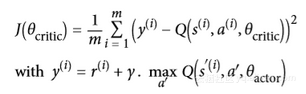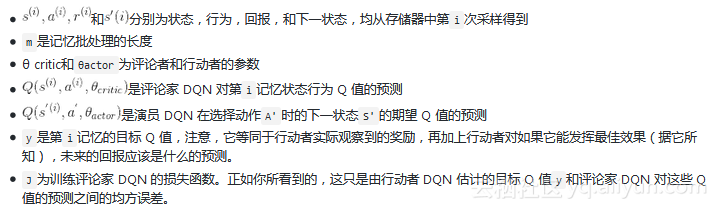X_action = tf.placeholder(tf.int32, shape=[None])
q_value = tf.reduce_sum(critic_q_values * tf.one_hot(X_action, n_outputs), axis=1, keep_dims=True)


y = tf.placeholder(tf.float32, shape=[None, 1])
cost = tf.reduce_mean(tf.square(y - q_value))
global_step = tf.Variable(0, trainable=False, name='global_step')
training_op = optimizer.minimize(cost, global_step=global_step)
init = tf.global_variables_initializer()
saver = tf.train.Saver()


from collections import deque

replay_memory_size = 10000
replay_memory = deque([], maxlen=replay_memory_size)

def sample_memories(batch_size):
indices = rnd.permutation(len(replay_memory))[:batch_size]
cols = [[], [], [], [], []] # state, action, reward, next_state, continue
for idx in indices:
memory = replay_memory[idx]
for col, value in zip(cols, memory):
col.append(value)
cols = [np.array(col) for col in cols]
return (cols, cols, cols.reshape(-1, 1), cols,cols.reshape(-1, 1))


eps_min = 0.05
eps_max = 1.0
eps_decay_steps = 50000
def epsilon_greedy(q_values, step):
epsilon = max(eps_min, eps_max - (eps_max-eps_min) * step/eps_decay_steps)
if rnd.rand() < epsilon:
return rnd.randint(n_outputs) # 随机动作
else:
return np.argmax(q_values) # 最优动作


n_steps = 100000  # 总的训练步长
training_start = 1000  # 在游戏1000次迭代后开始训练
training_interval = 3  # 每3次迭代训练一次
save_steps = 50  # 每50训练步长保存模型
copy_steps = 25  # 每25训练步长后复制评论家Q值到行动者
discount_rate = 0.95
skip_start = 90  # 跳过游戏开始(只是等待时间)
batch_size = 50
iteration = 0  # 游戏迭代
checkpoint_path = "./my_dqn.ckpt"
done = True # env 需要被重置


with tf.Session() as sess:
if os.path.isfile(checkpoint_path):
saver.restore(sess, checkpoint_path)
else:
init.run()
while True:
step = global_step.eval()
if step >= n_steps:
break
iteration += 1
if done: # 游戏结束，重来
obs = env.reset()
for skip in range(skip_start): # 跳过游戏开头
obs, reward, done, info = env.step(0)
state = preprocess_observation(obs)

# 行动者评估要干什么
q_values = actor_q_values.eval(feed_dict={X_state: [state]})
action = epsilon_greedy(q_values, step)

# 行动者开始玩游戏
obs, reward, done, info = env.step(action)
next_state = preprocess_observation(obs)

# 让我们记下来刚才发生了啥
replay_memory.append((state, action, reward, next_state, 1.0 - done))        state = next_state

if iteration < training_start or iteration % training_interval != 0:                continue

# 评论家学习
X_state_val, X_action_val, rewards, X_next_state_val, continues = (            sample_memories(batch_size))
next_q_values = actor_q_values.eval( feed_dict={X_state: X_next_state_val})
max_next_q_values = np.max(next_q_values, axis=1, keepdims=True)
y_val = rewards + continues * discount_rate * max_next_q_values
training_op.run(feed_dict={X_state: X_state_val,X_action: X_action_val, y: y_val})

# 复制评论家Q值到行动者
if step % copy_steps == 0:
copy_critic_to_actor.run()

# 保存模型
if step % save_steps == 0:
saver.save(sess, checkpoint_path)【翻译】Sklearn 与 TensorFlow 机器学习实用指南 —— 第11章 训练深层神经网络（上）

984 0【翻译】Sklearn与TensorFlow机器学习实用指南 ——第12章 设备和服务器上的分布式TensorFlow（下）

768 0【翻译】Sklearn与TensorFlow机器学习实用指南 ——第12章 设备和服务器上的分布式TensorFlow（上）

997 0【翻译】Sklearn与TensorFlow机器学习实用指南 ——第12章 设备和服务器上的分布式TensorFlow（下）
1414 0【翻译】Sklearn 与 TensorFlow 机器学习实用指南 —— 第11章 训练深层神经网络（中）
1590 0【翻译】Sklearn 与 TensorFlow 机器学习实用指南 —— 第11章 训练深层神经网络（上）
3267 0【翻译】Sklearn 与 TensorFlow 机器学习实用指南 —— 第10章 人工神经网络介绍（下）
1660 0《Sklearn 与 TensorFlow 机器学习实用指南》 第6章 决策树

1170 0《Scikit-Learn与TensorFlow机器学习实用指南》第16章 强化学习

2472 0《Scikit-Learn与TensorFlow机器学习实用指南》第8章 降维

1796 0

7194

9

+ 订阅